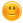# For each breath that you take, how many of the air molecules would also have been breathed by Isaac Newton.

+15 votes
8,433 views
asked Mar 8, 2013 in Science
)during his life time, the atmosphere is about 8 km high, and the molecules in the air each occupy a space representing a little cubic box about 3.33*10^-9 m along a side, the earths radius is 6..38*10^6. make any reasonable assumptions for any data needed.

## 5 Answers

0 votes
answered Apr 24 by (44,660 points)
you need to calculate
0 votes
answered May 20, 2016 by (425,340 points)
615360000 is that number
+1 vote
answered Mar 29, 2013 by (33,010 points)
I do not know you would have to calculate the air for a long time!
+2 votes
answered Mar 12, 2013 by (740 points)
6*10^12 is the amount of air
+2 votes
answered Mar 10, 2013 by (66,600 points)

Wow, that is a real tough one! You can figure out how much air Newton breathed in during his lifetime and convert that to Liters and then to cubic meters. After that you can get the number of L of atmosphere and figure out the fraction of air Newton breathed assuming perfect mixing of his breaths. Once you have that you need to calculate the number molecules per breath of air, so you would factor in the air density and the number of molecules in a Liter (Avogadros numbers). You can then estimate the number molecules we take in per breath.

Kudos if you can figure it out. I'm sure it is a postive value, which is really neat to think about - We are all breathing the same molecules every time we take a breath of one the greatest scientists of all time